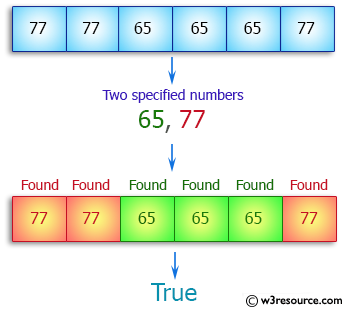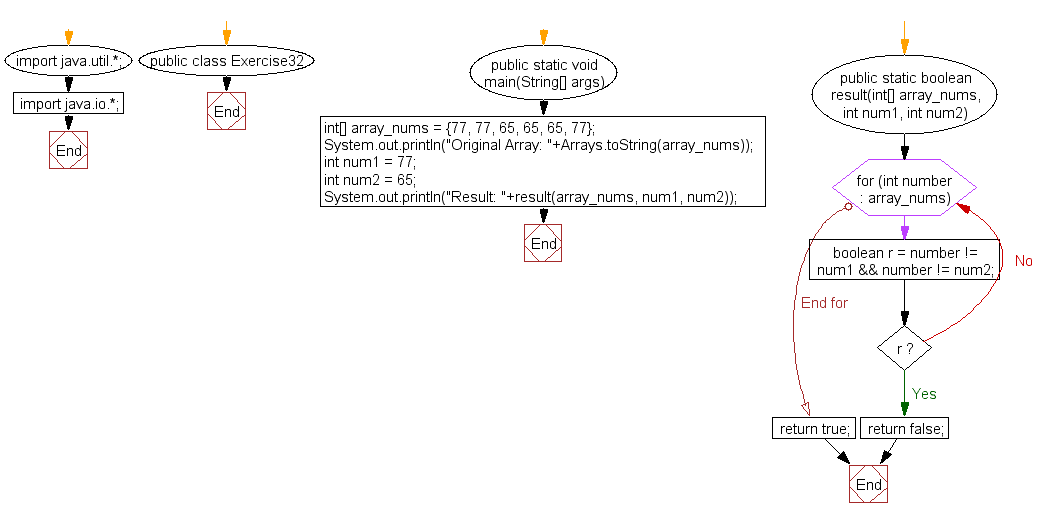﻿ Java: Check if an array of integers contains two elements# Java Array Exercises: Check if an array of integers contains two specified elements

## Java Array: Exercise-32 with Solution

Write a Java program to check if an array of integers contains two specified elements 65 and 77.

Pictorial Presentation:Sample Solution:

Java Code:

``````import java.util.*;
import java.io.*;
public class Exercise32 {
public static void main(String[] args)
{
int[] array_nums = {77, 77, 65, 65, 65, 77};
System.out.println("Original Array: "+Arrays.toString(array_nums));
int num1 = 77;
int num2 = 65;

System.out.println("Result: "+result(array_nums, num1, num2));
}

public static boolean result(int[] array_nums, int num1, int num2) {
for (int number : array_nums) {
boolean r = number != num1 && number != num2;
if (r) {
return false;
}
}
return true;
}
}
```
```

Sample Output:

```
Original Array: [77, 77, 65, 65, 65, 77]
Result: true
```

Flowchart:Visualize Java code execution (Python Tutor):

Java Code Editor:

Improve this sample solution and post your code through Disqus

What is the difficulty level of this exercise?

Test your Programming skills with w3resource's quiz.

﻿

## Java: Tips of the Day

getEnumMap

Converts to enum to Map where key is the name and value is Enum itself.

```public static <E extends Enum<E>> Map<String, E> getEnumMap(final Class<E> enumClass) {
return Arrays.stream(enumClass.getEnumConstants())
.collect(Collectors.toMap(Enum::name, Function.identity()));
}
```

Ref: https://bit.ly/3xXcFZt